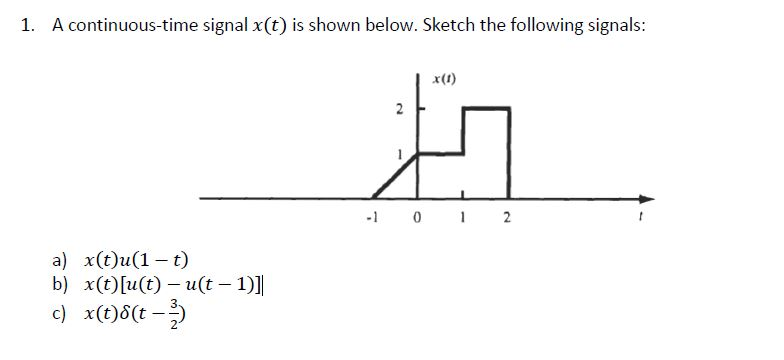### Create an Account

Home / Questions / A continuous-time signal x(t) is shown below. Sketch the following signals: 1 x) 2 0 2 a) x(t)u(1 t)

# A continuous-time signal x(t) is shown below. Sketch the following signals: 1 x) 2 0 2 a) x(t)u(1 t)A continuous-time signal x(t) is shown below. Sketch the following signals: 1 x) 2 0 2 a) x(t)u(1 t) b) x(t)u(t)u (t 1)] c) x(t)8(t

Apr 01 2020 View more View LessSubscribe To Get Solution